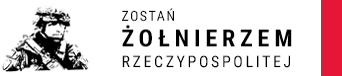## Cryptographic problems can be broken by quantum annealing

Is cryptography used, inter alia, will remain safe in banks when quantum computers come into play? The method that allows for the practical implementation of the attack with the use of quantum computing was proposed by Maj. dr inż. Michał Wroński from the Faculty of Cybernetics of the Military University of Technology and presented it during the International Conference on Computational Science 2021.

Many cryptographic algorithms are based on the problem of discrete logarithm on elliptic curves. So far, it has not been possible to break it in practice (even for very small cases) using quantum methods. For now, the ciphers are safe.

“We are still a long way from breaking the discrete logarithm on elliptic curves for the cases used in practice. But if this happens, then modern cryptography, even the one used in banks, will be vulnerable to attacks, primarily with the use of variants of the Shor algorithm. Therefore, intensive research is currently being carried out on algorithms that are also resistant to quantum computers "- explains the meaning of his research, Maj. dr inż. Michał Wroński.

A scientist from the Institute of Mathematics and Cryptology showed how the known attack on discrete logarithm on elliptic curves can be transformed into a form that can be solved by computers using quantum annealing (a kind of computation that brings very fast solutions)

The researcher admits that the implemented example is not large, but the quantum method presented by him has solved the biggest problem of the discrete logarithm on elliptic curves so far.

"Research on transforming cryptographic problems into problems solvable with the use of quantum annealing should be continued dynamically, because thanks to this, significant progress in this field of knowledge is possible" - emphasizes the author.

The article "Index Calculus Method for Solving Elliptic Curve Discrete Logarithm Problem Using Quantum Annealing" was published on June 16, 2021 in the conference materials of the International Conference on Computational Science 2021.